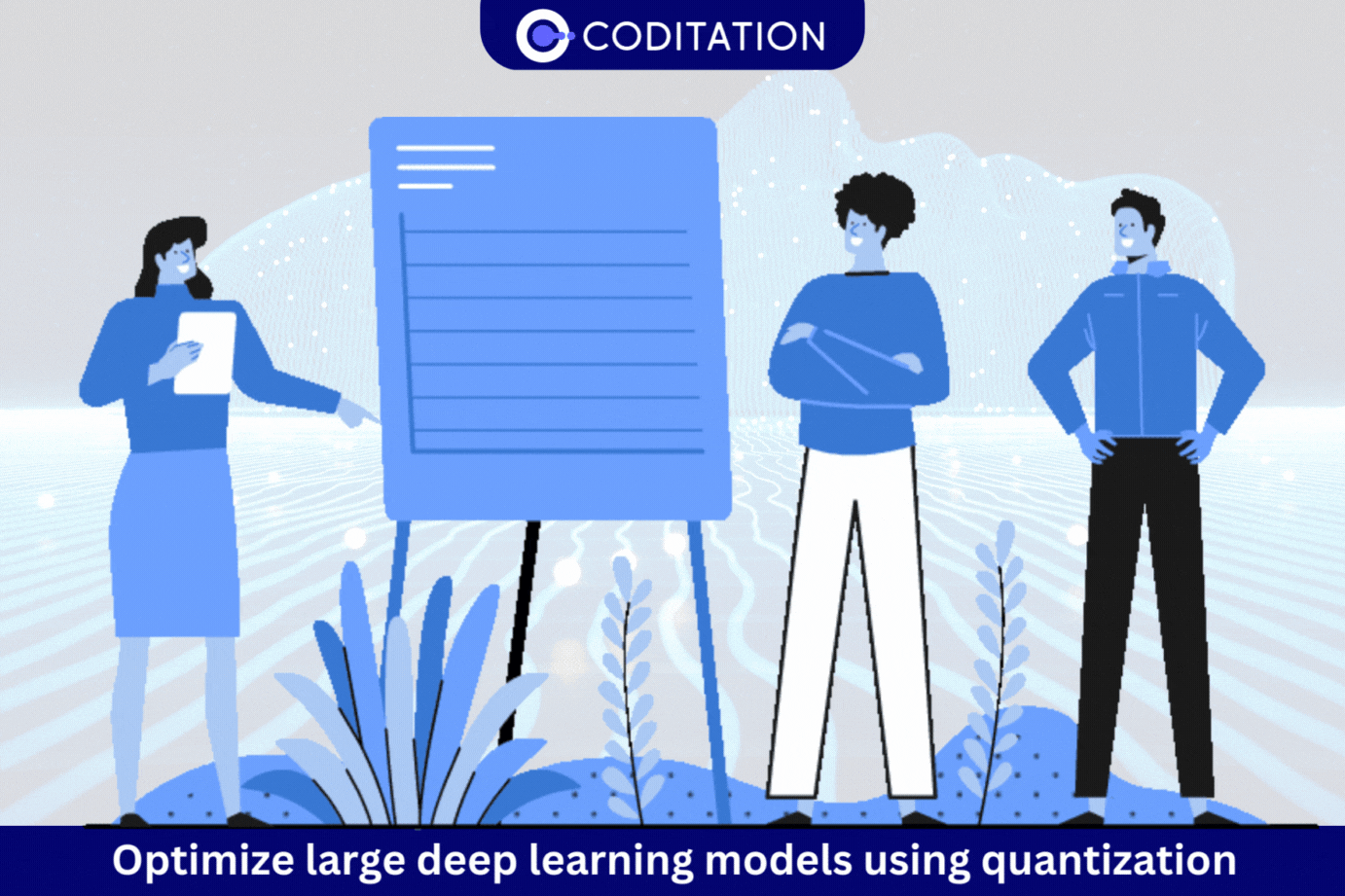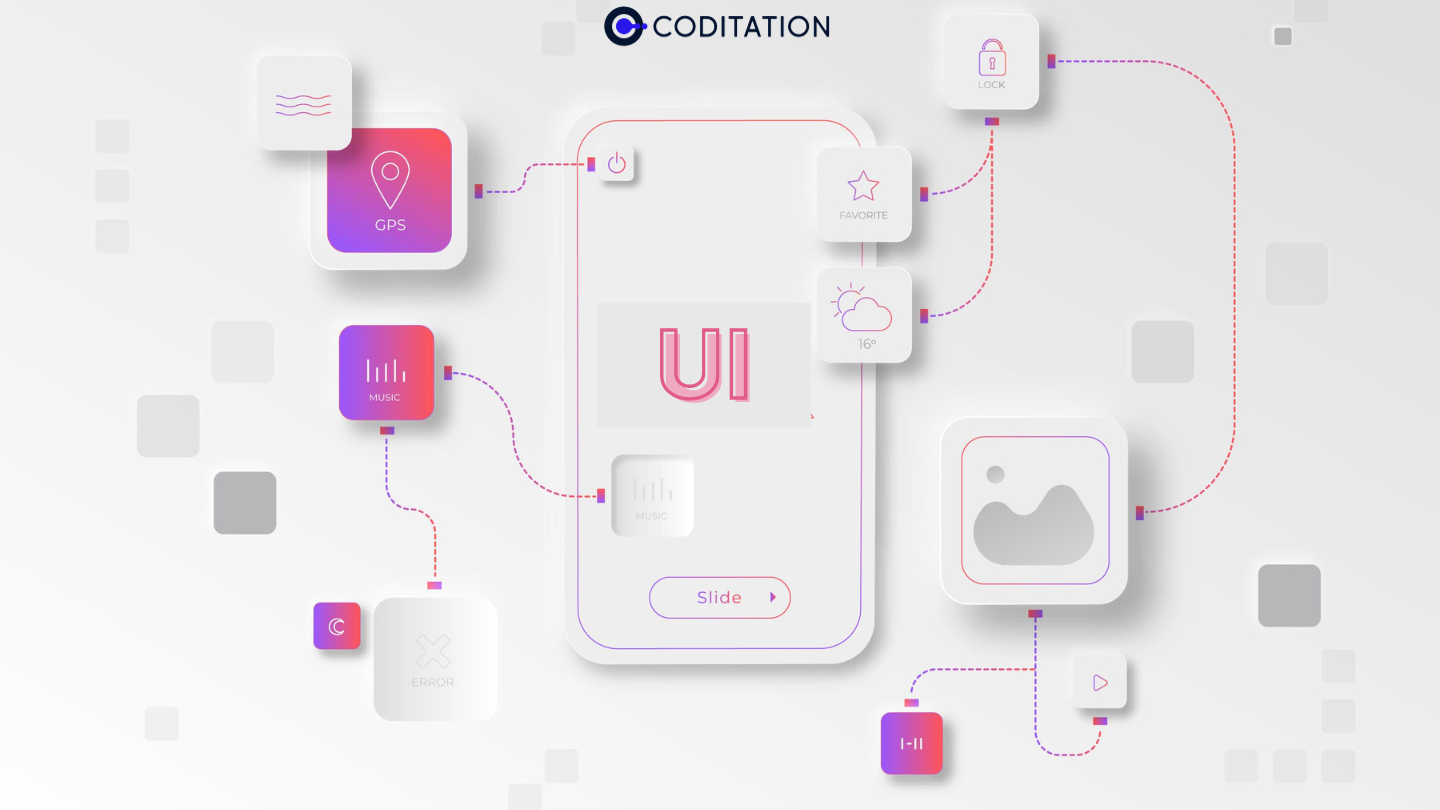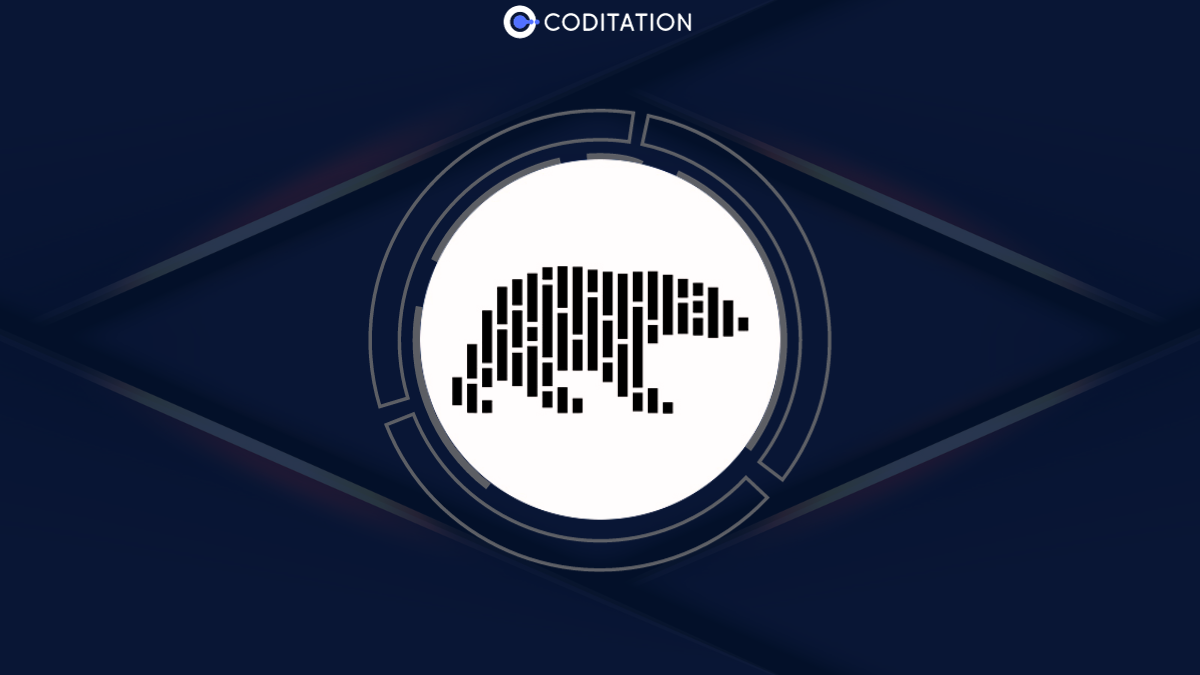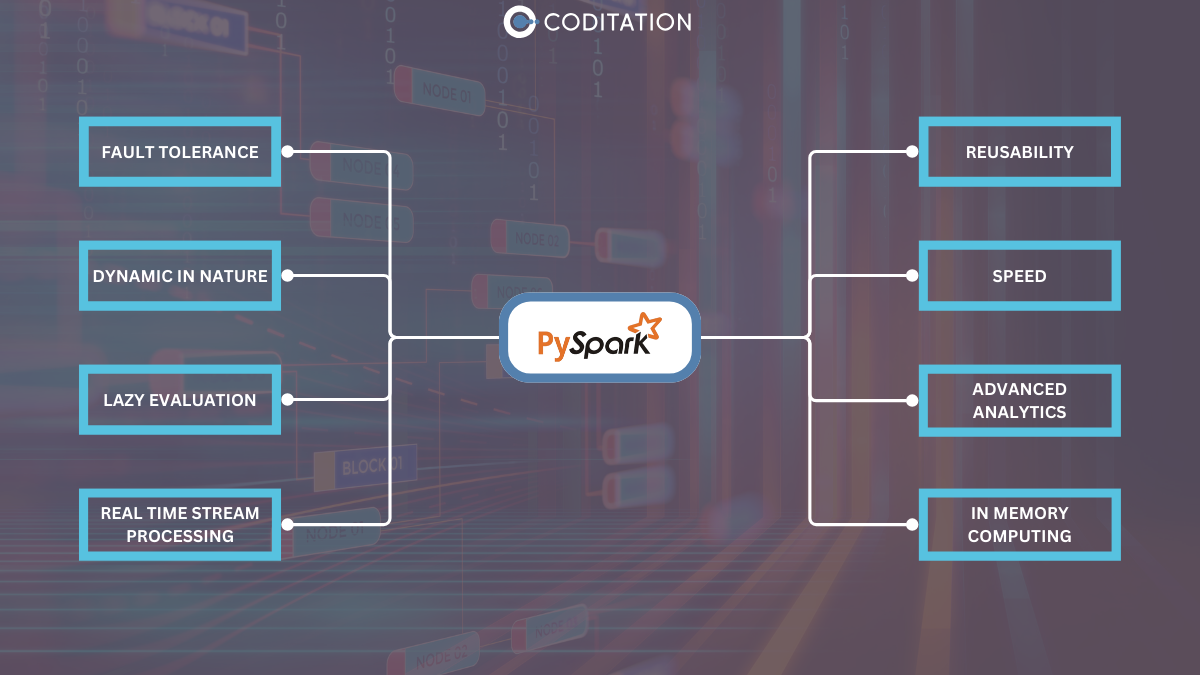# How to optimize large deep learning models using quantization

Quantization is the process of mapping continuous infinite values to a smaller set of discrete finite values. In this blog, we demonstrate optimization of large deep learning model using quantization.## How to optimize large deep learning models using quantization

Over the years, Convolutional Neural Networks (CNNs) have achieved remarkable success in various computer vision tasks such as image classification, object detection, and segmentation. However, when it comes to executing these complex models on mobile devices, challenges arise due to their size and computational requirements. Unfortunately, modern CNNs are not optimized for execution on mobile platforms, as they have been primarily evaluated based on classification and detection accuracy rather than model complexity and computational efficiency.

The growing demand for deploying CNNs on mobile platforms such as smartphones, AR/VR devices, and drones has created a need for smaller and more efficient models that can accommodate limited on-device memory and provide low latency for seamless user engagement. To address this need, a growing field of research has emerged, with a focus on reducing the model size and inference time of CNNs with minimal accuracy losses. This blog aims to start examining the optimization methods of CNNs to better suit mobile and embedded platforms and enable real-time performance with limited computational resources. In short, optimization is a crucial step in the development and deployment of deep learning models on mobile and embedded devices. It enables us to create models that are both accurate and efficient and opens up new possibilities for applications in fields such as healthcare, transportation, and augmented reality. Three common techniques used for model optimization are pruning, quantization, and topology optimization.

Pruning involves reducing the number of parameters in a model by eliminating redundant or less important weights. Quantization involves reducing the precision of the model's weights and activations, typically from floating-point numbers to integers. Topology optimization involves updating the original model architecture to a more efficient one with reduced parameters or faster execution.

Here the focus is particularly on playing with number representation ,i.e., Quantization.

#### Let’s learn quantization

Quantized models are those where we represent the models with lower precision, such as 8-bit integers as opposed to 32-bit float. Lower precision is a requirement to leverage certain hardware. It can significantly reduce memory and storage requirements, improve energy efficiency, compress the model size, adapt the model to different hardware platforms, and reduce the overall latency. It can be an important tool for deploying deep learning models in resource-constrained environments.

#### How to quantize

There are several ways to quantize a deep learning model. Some of the most common methods are:

1. Fixed-Point Quantization: This method involves representing weights and activations as fixed-point numbers with a specified number of integer and fractional bits. For example, 8-bit fixed-point quantization would represent each value using 1 sign bit, 6 integer bits, and 1 fractional bit. Fixed-point quantization is a simple and computationally efficient method.
2. Dynamic Fixed-Point Quantization: This method involves using different precision levels for different layers or activations in a deep learning model. This allows for more fine-grained control over the trade-off between accuracy and computational complexity.
3. Integer-Only Quantization: This method involves representing weights and activations as integers, without any fractional bits. This method is even simpler than fixed-point quantization and can be used for extremely low-power devices.
4. K-Means Clustering: This method involves grouping similar weights or activations and representing each group by its centroid value. This method can provide better accuracy and resolution than fixed-point quantization.
5. Quantization-Aware Training: This method involves training a deep learning model with quantization in mind. This allows the model to adapt to the limitations of quantization and achieve better accuracy with lower precision.
6. Hybrid Quantization: This method involves combining multiple quantization techniques to achieve the best possible balance between accuracy and computational complexity.

Overall, the choice of quantization method depends on the specific requirements of the deep learning model, including the size and complexity of the model, the available computational resources, and the desired level of accuracy and resolution. From all the above methods here I have presented 1st Part of the blog: detailing K-Means-based Quantization and Linear Quantization

##### 1. K-Means-based Quantization

K-Means-based quantization is a popular method for reducing the precision of weights and activations in a deep learning model to save memory and computation. The method works by clustering the weights or activations into a smaller set of centroids using the K-Means clustering algorithm. Each centroid represents a quantized value, and the original values are replaced by the nearest centroid.
For example, if we have a set of weights or activations with a range of [0, 1], and we want to quantize to 4 bits, we can use K-Means clustering to find 24=16 centroids that best represent the distribution of values. The nearest centroid value then replaces each weight or activation. The quantization error is the difference between the original value and the centroid value, which can be backpropagated during training to update the centroids. It is evident from the example below that the size of the original weight matrix reduces by a factor of 3.2.

K-Means-based quantization can achieve high compression rates with minimal loss in accuracy, as long as the number of centroids is chosen carefully to balance between compression and accuracy. It is also a simple and efficient method that can be easily implemented on a variety of hardware platforms. However, it may not work well for highly non-linear or sparse models and may require careful tuning of hyperparameters for best results.

Here is a sample Python code for K-Means quantization with test cases:

``````
import numpy as np
from sklearn.cluster import KMeans
def k_means_quantize(data, n_clusters):
# Convert data to a 2D array
data = np.array(data).reshape(-1, 1)

# Perform K-Means clustering
kmeans = KMeans(n_clusters=n_clusters).fit(data)

# Get the centroids
centroids = kmeans.cluster_centers_

# Quantize the data using the centroids
quantized_data = kmeans.predict(data)

# Convert the quantized data back to the original shape
quantized_data = quantized_data.reshape(data.shape)
return quantized_data, centroids
```
```

# Test case 1

data = [1, 3, 5, 7, 9]
n_clusters = 2
quantized_data, centroids = k_means_quantize(data, n_clusters)
print("Quantized data: ", quantized_data)
print("Centroids: ", centroids)

# Test case 2

data = [10, 20, 30, 40, 50, 60, 70, 80, 90]
n_clusters = 3
quantized_data, centroids = k_means_quantize(data, n_clusters)
print("Quantized data: ", quantized_data)
print("Centroids: ", centroids)

# Test case 3

data = [-10, -5, 0, 5, 10]
n_clusters = 2
quantized_data, centroids = k_means_quantize(data, n_clusters)
print("Quantized data: ", quantized_data)
print("Centroids: ", centroids)

In the test cases, we input some sample data and the number of clusters for K-Means clustering. The code then performs K-Means quantization on the data and returns the quantized data and the centroids of the clusters. We can print the results of each test case to verify that the code is working correctly.

Now let’s move on to the next technique linear quantization.

##### 2. Linear Quantization

Linear quantization is a technique used in deep learning models to reduce the bit-width of the weights and activations, to reduce the model size and computation cost. The quantization is linear in the sense that it maps the full range of floating-point values to a limited set of quantized values with a fixed distance between adjacent values. This fixed distance is determined by the number of bits used for quantization, and the range of the values being quantized. For example, quantizing to 8 bits with a range of [-1, 1] would map the range to 256 equidistant levels, each represented by a unique 8-bit binary code. The quantization can be done offline, during training, or online, during inference, depending on the requirements of the application. Linear quantization can result in some loss of accuracy compared to the full-precision floating-point values, but this can be mitigated by appropriate training techniques and quantization-aware optimizations.

Linear quantization directly rounds the floating-point value into the nearest quantized integer after range truncation and scaling. Linear quantization can be represented as

r=S(q-z)

where r is a floating point real number, q is an n-bit integer quantized number, Z is the quantization zero point and S is the quantization scaling factor. Both constant Z and S are quantization parameters. The flow of quantized-tensor computation is depicted as follows.

- From all the above formulations of linear quantization, Here's the Python code for linear quantization
Input: floating tensor to be quantized, bitwidth: quantization bit width,
Output
: scale: scaling factor, zero_point: the desired centroid of tensor values, quantized_tensor

``````
def linear_quantize(fp_tensor, bitwidth):

quantized_max = (1 << (bitwidth - 1)) - 1
quantized_min = -(1 << (bitwidth - 1))
fp_max = fp_tensor.max().item()
fp_min = fp_tensor.min().item()

############### calculating scale ###############
scale = (fp_max - fp_min) / (quantized_max - quantized_min)

############### calculating zero_point #################
zero_point = int(round(quantized_min - fp_min/scale))

# clip the zero_point to fall in [quantized_min, quantized_max]
if zero_point < quantized_min:
zero_point = quantized_min
elif zero_point> quantized_max:
zero_point = quantized_max
else: # convert from float to int using round()
zero_point = round(zero_point)
############### scale the fp_tensor ###############
scaled_tensor = torch.div(fp_tensor, scale)
############### round the floating value to integer value ###############
rounded_tensor = torch.round(scaled_tensor)
rounded_tensor = rounded_tensor.to(dtype)
############### shift the rounded_tensor to make zero_point 0 ###############
shifted_tensor =rounded_tensor + zero_point
############### clamp the shifted_tensor to lie in bitwidth-bit range#################

quantized_tensor = shifted_tensor.clamp_(quantized_min, quantized_max)
return quantized_tensor``````
##### `- The output of Linear Quantization`

`‍Summary:`

`‍The basic concept of neural network quantization: converting the weights and activations of neural networks into a limited discrete set of numbers. Linear quantization and clustering quantization are two popular techniques used in deep learning to reduce the precision of weights and activations in neural networks. Here's a summary of each technique:`

`1. Linear quantization: Linear quantization is a technique where the range of values for the weights and activations is divided into a fixed number of equally spaced intervals, and each interval is assigned a unique quantized value. This technique is simple and computationally efficient and can be easily implemented in hardware. However, it may not always result in the best accuracy, especially for models with complex or non-uniform distributions of values.2. Clustering quantization: Clustering quantization is a technique where the values of weights and activations are clustered into a smaller set of representative values, and the values are then quantized to the nearest representative value. This technique can result in better accuracy than linear quantization, especially for models with non-uniform distributions of values. Clustering quantization can be performed using various algorithms, such as k-means clustering or vector quantization, and can be customized based on the specific requirements of the model.`

`In summary, both linear quantization and clustering quantization are effective techniques for reducing the precision of weights and activations in neural networks. Linear quantization is simple and computationally efficient, while clustering quantization can result in better accuracy for models with non-uniform distributions of values. The choice of which technique to use depends on the specific requirements of the model and the hardware constraints of the deployment environment. Stay tuned as we will be further discussing the optimization techniques that can be applied to the large models in our upcoming blog post.`

`Hi, my name is Kiran Kamble. When I am done analyzing data, I play badminton and cricket and weekends are meant for hiking.`

## `Latest Articles`

`View All Articles`
`Understanding UI Frameworks, Theming, and Best Practices in React DevelopmentThis comprehensive blog explores the significance of UI frameworks, theming in React applications, popular UI frameworks such as MaterialUI, Bootstrap, and Ant Design, along with their strengths and weaknesses. It delves into the importance of theming for consistent UI/UX, provides insights into various theming approaches in React, and offers a step-by-step guide on implementing theming in React applications.8 min read`
`High-Performance Data Analysis with Polars: A Comprehensive GuideDiscover how Polars, a powerful Rust-based DataFrame library for Python, revolutionizes high-performance data analysis and manipulation. Explore its key features, from speed and efficiency to data manipulation capabilities and lazy evaluation.7 min read`
`Simplifying Data Processing with PySpark on Amazon EMR: Best Practices, Optimization, and SecurityIn this blog, we cover a wide range of topics, including monitoring, optimization, design patterns, error handling, security measures, scalability, and cost optimization, providing valuable insights and guidance for data engineers and practitioners working with big data processing on cloud platforms like Amazon EMR.8 min read`
``` window.onload = function() { Calendly.initBadgeWidget({ url: 'https://calendly.com/coditation/talk2expert?hide_event_type_details=1&hide_gdpr_banner=1&primary_color=551ff7', text: 'Book Your Meeting', color: '#5223f6', textColor: '#ffffff', branding: false }); } hljs.initHighlightingOnLoad();```
``` // define the load gist function var loadGist = function(elem) { var gistUrl = \$(elem).text().match(/\bhttps?:\/\/\S+(.js)/gi); if (typeof gistUrl === 'undefined' || gistUrl === null || gistUrl.length == 0) return; //load gist \$(elem).show(); \$(elem).empty(); postscribe(elem, '<script src=' + gistUrl+ '><\/script>'); }; if (postscribe) { var gists = \$('p:contains("CODE:")'); gists.hide(); gists.toArray().forEach(loadGist); } else { console.log("you need to include postscribe in your site for this to work") } ```